Math Concepts
Complete Guide: How to divide two numbers using Abacus?
0

 1 Introduction 2 A Brief Journey Of Abacus through time 3 How to count numbers on Abacus? 4 How to perform division on Abacus? 5 Summary 6 FAQs 7 External References

13 November  2020

## Introduction

Have you ever wondered how people managed without an important device like Calculators centuries ago?

What if I tell you that they had a calculator all along!

Yes, it was the abacus that was heavily used for complex calculations before the invention of electronic calculators.

Abacus is a useful learning piece of equipment for the visually impaired, as well as for anyone who wants to learn the origins of a modern calculator.

Just by moving the beads from left to right, you can add up to a billion or more.

Abacus is a tool that can be used to calculate — Add, Subtract, Multiply & divide, apart from being able to do other arithmetic functions and Brain activation.

In this article, you will learn how Abacus is used for simple division. Download the PDF below to know the history of the abacus and division using an abacus.

## A Brief Journey Of Abacus through time

According to written text, Counting tables have been used for over 2000 years dating back to Greeks and Romans.

The “normal method of calculation in Ancient Greece and Rome was done by moving counters on a smooth board or table suitably marked with lines or symbols to show the ‘places.’

The origin of the portable bead frame abacus is not well-known. It was thought to have originated out of necessity for travelling merchants.

Some historians give the Chinese credit as the inventors of bead frame abacus, while others believe that the Romans introduced the abacus to the Chinese through trade.

Today the abacus lives in rural parts of Asia and Africa and has proven to be a handy computing tool.

The widely used abacus throughout China and other parts of Asia is Known as Suanpan. It has five unit beads on each lower rod and two ‘five beads on each upper rod.

The modern Japanese abacus, known as a Soroban, was developed from the Chinese Suan-pan.

The Russian abacus, the Schoty, has ten beads per rod and no dividing bar.

The Soroban abacus is considered ideal for the base-ten numbering system, in which each rod acts as a placeholder and can represent values 0 through 9.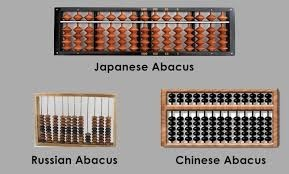The abacus has played a vital role in mathematics that can still be seen today.

The abacus is portable to be used by merchants and allows it to be introduced to all parts of the world. It is also flexible to be used across cultures.

## How to count numbers on Abacus?

On each rod, the Soroban abacus has one bead in the upper deck, known as the heaven bead, and four beads in the lower deck, known as the earth beads.

Each heaven bead in the upper deck has a value of 5; each earth bead in the lower deck has a value of 1.

Once it is understood how to count using an abacus, it is straightforward to find any integer for the user.

There are two general rules to solve any addition and subtraction problem with the Soroban abacus.

• The operator should always solve problems from left to right.
• The operator must be familiar with how to find complementary numbers, specifically, always with respect to 10

The value added to the original number to make 10 is the number’s complement.

For example, the complement of 7, with respect to 10, is 3 and the complement of 6, with respect to 10, is 4.

Consider adding 8 and 4. The process begins by registering 4 on the unit rod H,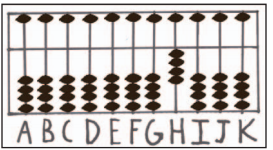Because the sum of the two numbers is greater than 9, subtraction must be used.

We subtract the complement of 8 - namely 2 - from 4 on rod H and add 1 bead to tens rod G.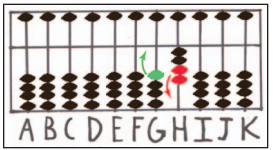This leaves us with 1 bead registered on rod G (the tens rod) and 2 beads on rod H (the unit rod)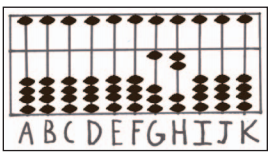This rule remains the same regardless of the numbers used. As we all know, subtraction is the opposite operation of addition.

Thus, when subtracting with the Soroban abacus, we add the complement and subtract 1 bead from the next highest place value.

## How to perform division on Abacus?

Solving division problems on the Soroban abacus mirrors familiar paper-and-pencil calculations. Furthermore, the process uses addition, subtraction, and multiplication.

The division is done by dividing one number in the divisor into one or possibly two numbers of the dividend at a time.

The operator multiplies after each division step and subtracts the product. The next part of the dividend is then tacked onto the remainder, and the process continues. It is more the same as doing it with a pencil and paper.

Below is a technique for working with division problems with four or more digits in the equation on the abacus.

When setting up problems of division on the abacus, the dividend is set on the right and the divisor is set on the left.

The common practice is followed by leaving 4 unused rods in between the two numbers. It’s on these unused rods where the quotient answer is formed.

So, let's start by reviewing the standard paper method.

• Find the starting column for the quotient. This will be the *first* position *n* where the number formed in columns 1 through *n* of the dividend is greater than or equal to the divisor.
• Columns *1* through *n* are called the *working digits*; column *n* is called the *current quotient column*.
•  Using approximation, figure out the *largest* number *i* such that *i* times the divisor is less than or equal to the number formed by the working digits.
• Write *i* in the current quotient column, and subtract *i* times the divisor from the working digits. The result *should be* a number *smaller* than the divisor. This is the *working remainder*.
• Copy digits to the right of the working digits, and append them to the working remainder from step 3, until you get a number *greater than or equal to* the divisor.
• The working remainder + the copied digits become the new working digits. The last column that you copied is the new *current quotient column*.
• If there are any blank spaces between the old and new current quotient columns, fill them with zeros.
• Go back to step 2, using the new working digits and current quotient column, until either the working remainder is zero.

The way we do it on the abacus is very similar to the way we did it on paper. The one difference is that on paper, we did the multiplication and subtraction as two steps; on the abacus, we're going to combine.

Let’s see some examples:

 Example 1

951 ÷ 3 = 317

In this example, the dividend has three whole numbers.

• Choose rod F as the unit and count three rods to the left.
• The divisor has one whole number so count one plus two back to the right. Set the first number of the dividend on rod F.

Step 1: Set the dividend 951 on rods FGH and the divisor 3 on A.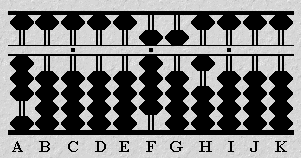Step 2: The divisor (3 on A) is smaller than the dividend (9 on F). Therefore apply "Rule I" and set the first number in the quotient two rods to the left on D. Divide 3 on A into 9 on F and set the quotient 3 on rod D.

Multiply the quotient 3 by 3 on A and subtract the product 9 from rod F. This leaves the partial quotient 3 on D and the remainder of the dividend 51 on rods GH.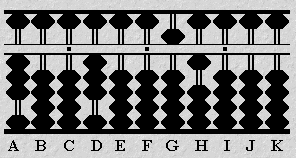Step 3: Divide 3 on A into 5 on G. Once again the divisor is smaller than the dividend so follow "Rule1". Set the quotient 1 on rod E.

Multiply the quotient 1 by 3 on A and subtract the product 3 from 5 on rod G. This leaves the partial quotient 31 on DE and the remainder of the dividend 21 on rods GH.Step 4: Divide 3 on A into 21 on GH and set the quotient 7 on rod F.

Multiply the quotient 7 by 3 on A and subtract the product 21 from rods GH leaving the answer 317 on rods DEF.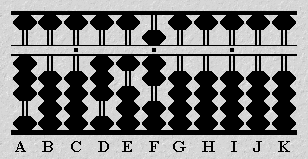So the answer we got after dividing on an abacus is  951 ÷ 3 = 317.

In problems of division, getting to the correct answer can sometimes involve a little trial and error. The division problem shown below is a case in point.

In this type of problem making a judgment as to what the exact quotient will be can be difficult.

Estimation is often the best course of action. It's the ease with which an operator can revise an incorrect answer that makes the soroban such a superior tool for solving problems of division.

 Example 2

0.14 ÷ 1.6 = 0.0875

• Choose D as the unit rod.
• In this example, because there are no whole numbers in the dividend there is no need to count to the left. The divisor has one whole number.
• Starting on rod D, count one plus two to the right. Set the first number of the dividend on rod G.

Step 1: Set the dividend 14 on rods GH and divisor 16 on rods AB.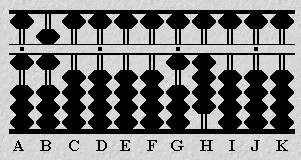Step 2: In this problem, the first two digits in the divisor are larger than those of the dividend.

Apply "Rule II". Set the first number in the quotient one rod to the left of the dividend, in this case on rod F. Think of the problem as dividing 16 on AB into 140 on GHI. Now estimate the quotient.

It seems reasonable to estimate a quotient of 7. Set 7 next to the dividend on rod F.

Multiply the quotient 7 by 1 on A and subtract the product 7 from 14 on rods GH.

Multiply the quotient 7 by 6 on B and subtract the product 42 from rods HI.

Revise both the quotient and the dividend. Add 1 to the quotient on rod F to make it 8. Subtract a further 16 from rods HI. This leaves the partial quotient 0.08 on CDEF and the remainder 12 on HI.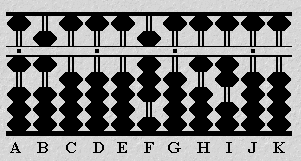Step 3: Borrow a zero from rod J and think of the problem as dividing 16 on AB into 120 on HIJ. Estimate a quotient of 8. Set 8 on rod G.

Multiply the quotient 8 by 1 on A and subtract the product 8 from 12 on rods HI.

Multiply the quotient 8 by 6 on B and subtract the product 48 from rods IJ.

Revise both the quotient and the dividend. Subtract 1 from 8 on G. Add 1 to 4 on the rod I.

Continue with the problem but this time use the revised quotient of 7. Multiply 7 on G by 6 on B and subtract 42 from rods IJ. This leaves the partial quotient 0.087 on rods CDEFG and the remainder, 8, on rod J.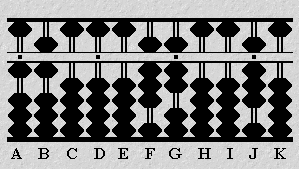Step 4: Borrow a zero from rod K and think of the problem as dividing 16 on AB into 80 on JK. Estimate a quotient of 5. Set 5 on rod H

Multiply the quotient 5 by 1 on A and subtract the product 5 from 8 on rod J.

Multiply the quotient 5 by 6 on B and subtract the product 30 from rods JK leaving 875 on rods FGH. With rod D acting as the unit, the answer reads 0.0875 on rods CDEFGH.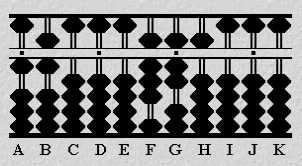So the answer we get after dividing on an abacus is: 0.14 ÷ 1.6 = 0.0875

## Summary

Division on the abacus to be really interesting. It's impressive how fast you can do division on such a trivial addition-based device.

Just an afternoon practising, and you can probably do most divisions on an abacus much faster than you could on paper.

The real trick to doing complicated things is exactly what we saw in the division: partitioning the abacus into regions.

Performing basic operations like the division on an abacus involves and develops a child's mind.

So, let’s recapitulate

• The abacus instrument is durable
• The abacus can be used to help young children learn numerical concepts.
• It helps in developing the skills at correctly manipulating beads on the counting tool.
• It builds an understanding of mathematical processes such as division, multiplication, subtraction, and addition.

Below are some blogs related to Abacus that will develop your understanding further:

Cuemath, student-friendly mathematics and coding platform, conducts regular Online Live Classes for academics and skill-development, and their Mental Math App, on both iOS and Android, is a one-stop solution for kids to develop multiple skills.

Check out the fee structure for all grades and book a trial class today!

## What is an abacus?

An Abacus is a manual aid for calculating which consists of beads that can be moved up and down on a series of sticks or strings within a usually wooden frame. The Abacus itself doesn't calculate; it's merely a device for helping a human being calculate by remembering what has been counted.

## Where was Abacus first used?

The Abacus (plural abaci or abacuses), also called a counting frame, is a calculating tool used in the ancient Near East, Europe, China, and Russia, centuries before the adoption of the written Arabic numeral system. The exact origin of the Abacus is still unknown.

## What are the advantages of learning how to use an abacus?

Mathematical skills lay a secure foundation for higher classes.
Abacus education improves the skills of

• Visualization (photographic memory)
• Concentration
• Listening Skills
• Memory, Speed
• Accuracy
• Creativity
• Self Confidence
• Self-Reliance resulting in Whole Brain Development

## How to use an abacus?

It is a simple device used for counting, much beneficial for the visually impaired, once you

know the basics of counting on an abacus, you can easily perform various operations on it .

Following are the basic steps to be followed:

• Assign each column a place value.
• Start counting with the beads in the lower row.
• Complete the "4/5 exchange.
• Repeat the pattern for the higher numbers.

## How to learn abacus?

We can easily learn abacus ,once we  learn the basics of how to count on abacus using beads and its placements  , we can perform basic operations on abacus.

Related Articles